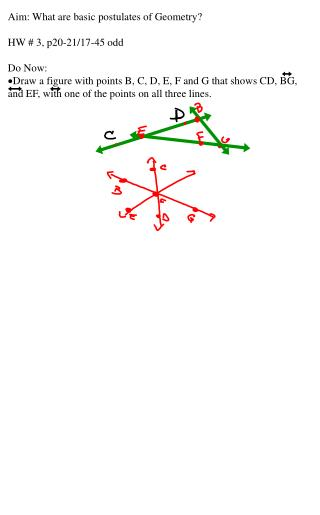DownloadDownload PresentationAim: What are basic postulates of Geometry? HW # 3, p20-21/17-45 odd Do Now:

# Aim: What are basic postulates of Geometry? HW # 3, p20-21/17-45 odd Do Now:

Download Presentation## Aim: What are basic postulates of Geometry? HW # 3, p20-21/17-45 odd Do Now:

- - - - - - - - - - - - - - - - - - - - - - - - - - - E N D - - - - - - - - - - - - - - - - - - - - - - - - - - -
##### Presentation Transcript

1. Aim: What are basic postulates of Geometry? • HW # 3, p20-21/17-45 odd • Do Now: • Draw a figure with points B, C, D, E, F and G that shows CD, BG, and EF, with one of the points on all three lines.

2. Complete the sentence. If two lines intersect, they intersect at exactly one ___________. This is a fact we have come to accept.

3. A postulate or axiom is an accepted statement of fact. Postulate 1-1 Through any two points there is exactly one line.

4. Postulate 1-2 If two lines intersect, then they intersect in exactly one point. Postulate 1-3 If two planes intersect, then they intersect in exacly one line. Postulate 1-4 Through any three noncollinear points there is exactly one plane.

5. Finding the intersection of two planes. What is the intersection of plane HGF and BCG? Name the two planes that intersect BF. What is the intersection of plane HGC and AED?

6. Identifying Planes Shade the plane that contains A, B and C Shade the plane that contains F, B and C Shade the plane that contains E, H and C Not all planes are the surfaces of the cube!